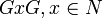# Supercharacter theory of an algebra group

Suppose$F$ is a finite field of size$q$, a prime power with underlying prime$p$. Suppose$N$ is a nilpotent associative finite-dimensional algebra over$F$. Suppose, further, that$G$ is the algebra group corresponding to$N$, i.e.,$G = 1 + N$..
The supercharacter theory of$G$ is a supercharacter theory defined as follows.
Note that$G$ is a subgroup of the unitization$N + F$, hence acts on$N + F$ by both left and right multiplication. The ideal$N$ is invariant under both actions, so we get a left and a right$G$-action on$N$ that commute with each other. Consider the double orbits of$N$ under these actions, i.e., sets of the form$GxG, x \in N$. The corresponding sets$1 + GxG$, which are subsets of$G$, are the superconjugacy classes.
Note that the conjugation action of$G$ on$N$ corresponds to its conjugation action on itself, and the orbits for this conjugation action are contained inside the double orbits, so the superconjugacy classes are unions of conjugacy classes.